# Triangle ABC

In a triangle ABC side b measure 10 cm less than the side a and side b is half of the side c. Calculate the length of sides if the circumference of the triangle is 42 cm.

a =  18
b =  8
c =  16

### Step-by-step explanation:

a+b+c=42
b = a-10
b = c/2

a+b+c = 42
a-b = 10
2b-c = 0

a = 18
b = 8
c = 16

Our linear equations calculator calculates it.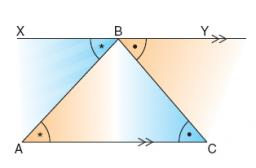Did you find an error or inaccuracy? Feel free to write us. Thank you!Tips to related online calculators
Do you have a system of equations and looking for calculator system of linear equations?

#### You need to know the following knowledge to solve this word math problem:

We encourage you to watch this tutorial video on this math problem:

## Related math problems and questions:

• Three sidesSide b is 2 cm longer than side c, side a is 9 cm shorter than side b. The triangle circumference is 40 cm. Find the length of sides a, b, c . .. .
• Sides of triangleTriangle has circumference 42 cm. Side a is 2 times shorter than side b and sice c is 2 cm longer than side a. Determine the sizes of sides of a triangle.
• DiamondThe diamond has a circumference of 48cm. Calculate the length of its side in dm.
• Sides of triangle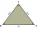Triangle circumference with two identical sides is 117cm. The third side measures 44cm. How many cms do you measure one of the same sides?
• RectangleThe length of one side of the rectangle is three times the length of the second side. What are the dimensions of the rectangle if its circumference 96 cm?
• Triangle - is RT?Triangle has a circumference of 90 cm. Side b is 1 cm longer than c, and side c is 31 cm longer than side a. Calculate the length of sides and determine whether a triangle is a right triangle.
• TrianglesEquilateral triangle with side 40 cm has the same perimeter as an isosceles triangle with arm of 45 cm. Calculate the base x of an isosceles triangle.
• Second sideCalculate the length of the other side of the rectangle if its circumference is 60 cm and one side is 10 cm long.
• Ratio of sidesThe triangle has a circumference of 21 cm, and the length of its sides is in a ratio of 6: 5: 3. Find the length of the longest side of the triangle in cm.
• Table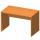Find the circumference of the table, where the long side is 1.28 meters and the short side is 86 cm.
• Parallelogram perimeter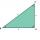The ABC triangle with sides a = 5cm, b = 3cm, c = 40mm has the center of the sides of the K,L,M. How many cm have the KBLM parallelogram perimeter?
• MidpointsTriangle whose sides are midpoints of sides of triangle ABC has a perimeter 45. How long is perimeter of triangle ABC?
• RectanglesHow many different rectangles with sides integers (in mm) have a circumference exactly 1000 cm? (a rectangle with sides of 50cm and 450cm is considered to be the same as a rectangle with sides of 450cm and 50cm)
• Medians in RT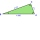The rectangular triangle ABC has a length of 10 cm and 24 cm. Points P, Q, R are the centers of the sides of this triangle. The perimeter of the PQR triangle is:
• TriangleProve whether you can construct a triangle ABC, if a=9 cm, b=6 cm, c=10 cm.
• Square gridSquare grid consists of a square with sides of length 1 cm. Draw in it at least three different patterns such that each had a content of 6 cm2 and circumference 12 cm and that their sides is in square grid.
• Similar trianglesTriangle A'B'C 'is similar to triangle ABC, whose sides are 5 cm, 8 cm, and 7 cm long. What is the length of the sides of the triangle A'B'C' if its circumference is 80 cm?